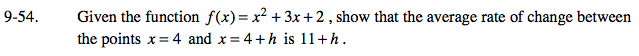### Home > PC > Chapter 9 > Lesson 9.1.4 > Problem9-54

9-54.

Given the function f(x) = x2 + 3x + 2, show that the average rate of change between the points x = 4 and x = 4 + h is 11 + h. Homework Help ✎$\frac{f(4+h)-f(4)}{4+h-4} =\frac{(4+h)^2+3(4+h)+2-(4^2+(3)(4)+2)}{4+h-4}=?$